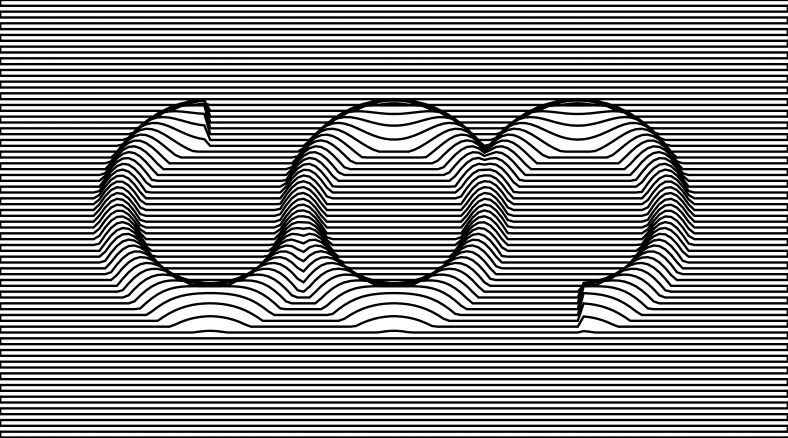# mathr / blog / #

## ReCode Project - Topographic Form animatedAfter I posted topographic form to the #diagrams IRC channel, I got a comment:

< luite_> ClaudiusMaximus: have you tried making an animation of that?

So that's what I did today, with this Haskell code:

import Diagrams.Prelude
import Diagrams.Backend.SVG.CmdLine (defaultMain)

import System.Environment (withArgs)

main = forM_ [0..49] $\f -> withArgs ["-w", "788", "-o", drop 1$ show (f + 100) ++ ".svg"] $defaultMain (diagram (2 * fromIntegral f / 50) (fromIntegral (if f >= 25 then 50 - f else f) / 25)) diagram r s = fromVertices (zigzag r s) # withEnvelope' (fromVertices [p2 (0, 3), p2 (135, 78)]) # bg white withEnvelope' a b = withEnvelope (a asTypeOf b) b zigzag r s = concat$ zipWith (line r (pi/2 * s)) ys (cycle [xs, reverse xs])

line r s y = map (point s (r + y))

point s y x = p2 (x, y + heightMap s x y)

heightMap s x y = maximum
[ arcH 36   (\t -> t < 0 || pi/2 < t - s) x y
, arcH 67.5 (\t -> True)                  x y
, arcH 99   (\t -> -pi/2 < t - s)         x y
]

arcH x0 f x y
| f t && ri < r && r < ro = h0 * cos (pi * (r - r0) / (ro - ri))
| otherwise = 0
where
t = atan2 y' x'
r = sqrt $x'^2 + y'^2 x' = x - x0 y' = y - y0 y0 = 40.5 r0 = 15.75 ri = r0 * 0.75 ro = r0 * 1.25 h0 = (ro - ri) / 2 xs = [0 .. 135] ys = [0 .. 81]  To postprocess the output into an animgif I did this in bash: for i in ??.svg do rsvg$i ${i%svg}png convert${i%svg}png \${i%svg}gif
done
gifsicle --delay 4 --loop --optimize ??.gif > out.gif